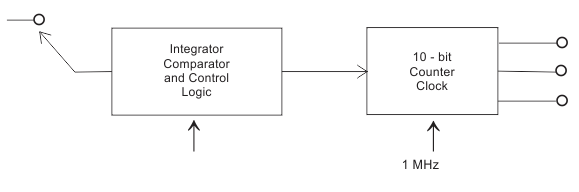# MCQs on Digital Electronics

##### Page 13 of 35. Go to page 1 2 3 4 5 6 7 8 9 10 11 12 13 14 15 16 17 18 19 20 21 22 23 24 25 26 27 28 29 30 31 32 33 34 35
01․ A flip flop is a _______ circuit.
Combinational
Sequential
Both A and B
None

As it saves its previous state...

02․ The simplified block diagram of a 10-bit A/D converter of dual slope integrator type is shown in figure. The 10-bit counter at the output is clocked by a 1 Mhz clock. Assuming negligible timing overhead for the control logic, the maximum frequency of the analog signal that can be converted using this A/D converted is approximately.
2 KHz.
1 KHz.
500 Hz.
250 Hz.

Maximum frequency of input in dual stop A/D converter is given as Tm = 2nTc. Where frequency of input and fc = 1/Tc = clock frequency. [math] f_m = rac{f_c}{2^n} = rac{10^6}{1024} = 1 \; KHz \; (approx).[/math]

03․ The effect of stray magnetic field on the actuating torque of a portable instrument is maximum when the operating field of the instrument and the stray fields are
Perpendicular.
Parallel.
Inclined at 60°.
Inclined at 30°.

Effect of stray magnetic field is maximum when the operating field and stray fields are parallel.

04․ A digital voltmeter has a read out range from 0 to 999 counts, the resolution is
1 V.
0.01 V.
1 mV.
1.1 V.

Resolution is the smallest change in the quantity being measured which will cause an observable change in output. In this case it is 1 mv.

05․ What is the difference between xor gate and exor gate.
xor and exor are complement of each other
xor gives two outputs while exor gives one
xor gives one output while exor gives two
both are same

Same name for one gate...

06․ One bit full adder has ________ inputs
2
3
4
any number

1. First bit 2. Second bit 3. Carry In

07․ One bit full adder has ________ outputs.
2
3
4
any number

1. Sum 2. Carry out

08․ If clock time period is 1ms, what is its frequency
1 mHz
1 kHz
1 MHz
None of the above

f = 1/T where f = frequency T = time period

09․ What is mean by FPU
floating point unit
floating parallel unit
floating point universal
floating parallel universal

It performs the arithmetic operations on floating point numbers...

10․ Which of these circuits have higher gate complexity 1. Carry look ahead adder 2. Ripple carry adder
1
2
both have same
none